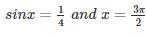# Engineering Math 5

## 10 Questions MCQ Test RRB JE for Computer Science Engineering | Engineering Math 5

Description
This mock test of Engineering Math 5 for Computer Science Engineering (CSE) helps you for every Computer Science Engineering (CSE) entrance exam. This contains 10 Multiple Choice Questions for Computer Science Engineering (CSE) Engineering Math 5 (mcq) to study with solutions a complete question bank. The solved questions answers in this Engineering Math 5 quiz give you a good mix of easy questions and tough questions. Computer Science Engineering (CSE) students definitely take this Engineering Math 5 exercise for a better result in the exam. You can find other Engineering Math 5 extra questions, long questions & short questions for Computer Science Engineering (CSE) on EduRev as well by searching above.
QUESTION: 1

### As xx varies from −1 to +3, which one of the following describes the behaviour of the function f(x) = x3–3x2 + 1?

Solution:

Given x various from –1 to +3
f(x) = x3 – 3x2 + 1

f′(x) = 3x2 – 6x

⇒ f′(−1) = 3(−1) 2 – 6 (−1) = 3 + 6 = 9 > 0,f′(1) = 3 – 6 < 0

f′(0) = 0,f′(2) = 3(4) – 6(2) = 0,

f′(3) = 3x2 – 6x = 3(a) – 6(3) = 0

∴ f(x) increases, then decreases and increases again

QUESTION: 2

### Given the following statements about a function f : R→R, select the right option: P: If f(x) is continuous at x = x0, then it is also differentiable at x = x0 Q: If f(x) is continuous at x = x0, then it may not be differentiable at x = x0 R: If f(x) is differentiable at x = x0, then it is also continuous at x = x0

Solution:

If the function is differentiable then always it is continuous but vice – versa is not true. If the function is continuous then it may (or) may not differentiable.

*Answer can only contain numeric values
QUESTION: 3

### Given f(x) = x3 + ax2 + bx + c and y-intercept for the function is 1. Also f has local extrema at x = -4 and x = 2. Then a + b + c = ____

Solution:

(i) As y-intercept is 1, c = 1

(ii) Also at x = −4,f′(x) = 0

3(−4)2+2a(−4)+b+1=0

48 – 8a + b + 1 = 0

49 – 8a + b = 0

(iii) Similarly 3(2)2 + 2a(2) + b + 1 = 0

12 + 4a + b + 1 = 0

13 + 4a + b = 0

∴ a = 3, b = -24

QUESTION: 4

Find C of Rolle’s theorem for f(x) = ex(sin x - cos x) in

Solution:

Given function f(x) = ex(sin x - cos x)

f(x) is continuous in 3π/4

f(x) is differentiable in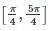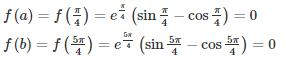f(a) = f(b)

According to Rolle’s theorem,

there exists at least one value C ∈ (a,b)

such that f’(c) = 0

f’(c) = 0

f’(x) = ex (sin x – cos x) + ex (cos x + sin x)

f’(c) = ec (sin c – cos c) + ec (cos c + sin c) = 0

⇒ 2 ec sin c = 0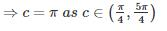QUESTION: 5

If f(x) is differentiable and g’(x) ≠ 0 such that f(1) = 4, f(2) = 16, f’(x)= 8g’(x) and g(2) = 4 then what is the value of g(1) ?

Solution:

By using Cauchy’s Mean value theorem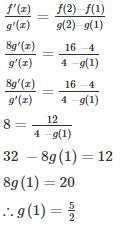QUESTION: 6

An open box is to be made out of square cardboard of 18 cm by cutting offs equal squares from the corners and turning up the sides. What is the maximum volume of the box in cm3?

Solution:

Let x be the corner side which is cut off and v be volume.
v = l × b × h

v = (18 − 2x) × (18 − 2x) × x

v = 4x3 − 72x2 + 324x

v′ = 12x− 144x + 324v′

v′′ = 24x − 144

v′ = 12x2 − 144x + 324 = 0

x2 − 12x + 27=0

(x−3) (x−9) = 0

(x=3) or (x = 9)

atx = 3

v′= 24x −144 = 24(3) −144 = −72<0

at x = 3 we have volume maximum

v = 4(3)3 − 72(2)2 + 324(2)

∴ v = 432

QUESTION: 7

What are the minimum and maximum value of the below-given function respectively?

f(x) = 3x3 − 9x2 − 27x + 30

Solution:

f(x) = 3x3 − 9x2 − 27x + 30

f′(x) = 9x2 − 18x − 27

f′′(x) = 18x − 18
Points at which maximum or minimum exists
f′(x) = 0

9x2 − 18x − 27 = 0

x2 − 2x − 3 = 0

(x − 3) (x + 1 ) = 0

(x = 3) or (x = −1)

Substitute the value in (x = 3) or (x = −1)

f′′(−1) = 18(−1) − 18 = − 36 < 0

∴ at x = −1,maximum value exists

f(−1) = 3(−1)−9(−1)−27(−1) + 30

f (−1) = 45

f′′ (3) = 18(3) − 18 = 36 > 0

∴ at x = 3, mimimum value exists

f(3) = 3(3)− 9(3)− 27(3) + 30

f (3) = −51

QUESTION: 8

Find the point at which maximum value of f(x) = 2x3−3x2 occurs in interval [-2, 2]?

Solution:

f(x) = 2x3 − 3x2

f′(x) = 6x2 − 6x

f′′(x) = 12x − 6

f′(x) = 6x2 − 6x = 0

6x (x−1) = 0

x = 0 or x = 1
Now check f(x) at x = 0, x = 1 and the end points of given interval since at end point tangent cannot be drawn so, above concept cannot be used to find maximum or minimum value for end points.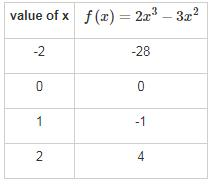QUESTION: 9

If f(x) = x3 − 3x−1 is continuous in the closed interval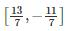and f’(x) exists in the open intervalthen find the value of c such that it lies inSolution:

Since given function is continuous and differentiable then by Lagrange’s Mean-Value Theorem.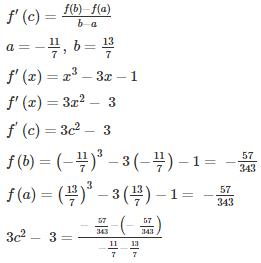3c2 − 3 = 0

c2 = 1

∴ c = ±1

QUESTION: 10Find the maximum and minimum values of f(x) = sin x + cos 2x where 0≤ x ≤2π

Solution:

f(x) = sin x + cos 2x in [0, 2π]

f’(x) = cos x – 2 sin 2x = cos x [1 – 4 sinx] = 0

⇒ cos x = 0; 1 – 4sinx = 0

⇒ x = π/2, 3π/2 in [0, 2π]

Sin x = 1/4, Also f’(x) exists for all x in [0, 2π]

Now f(0) = 1; f(2π) = 1; f(π/2) = 0; f(3π/2) = -2

At sinx = 1/4 , f(x) = 1/2 + (1 – 2(1/4)2) = 9/8

Therefore the maximum and minimum values of f(x) are 9/8 and -2 occurred at